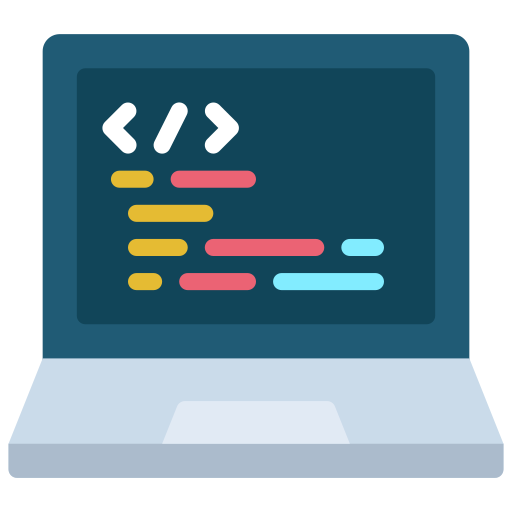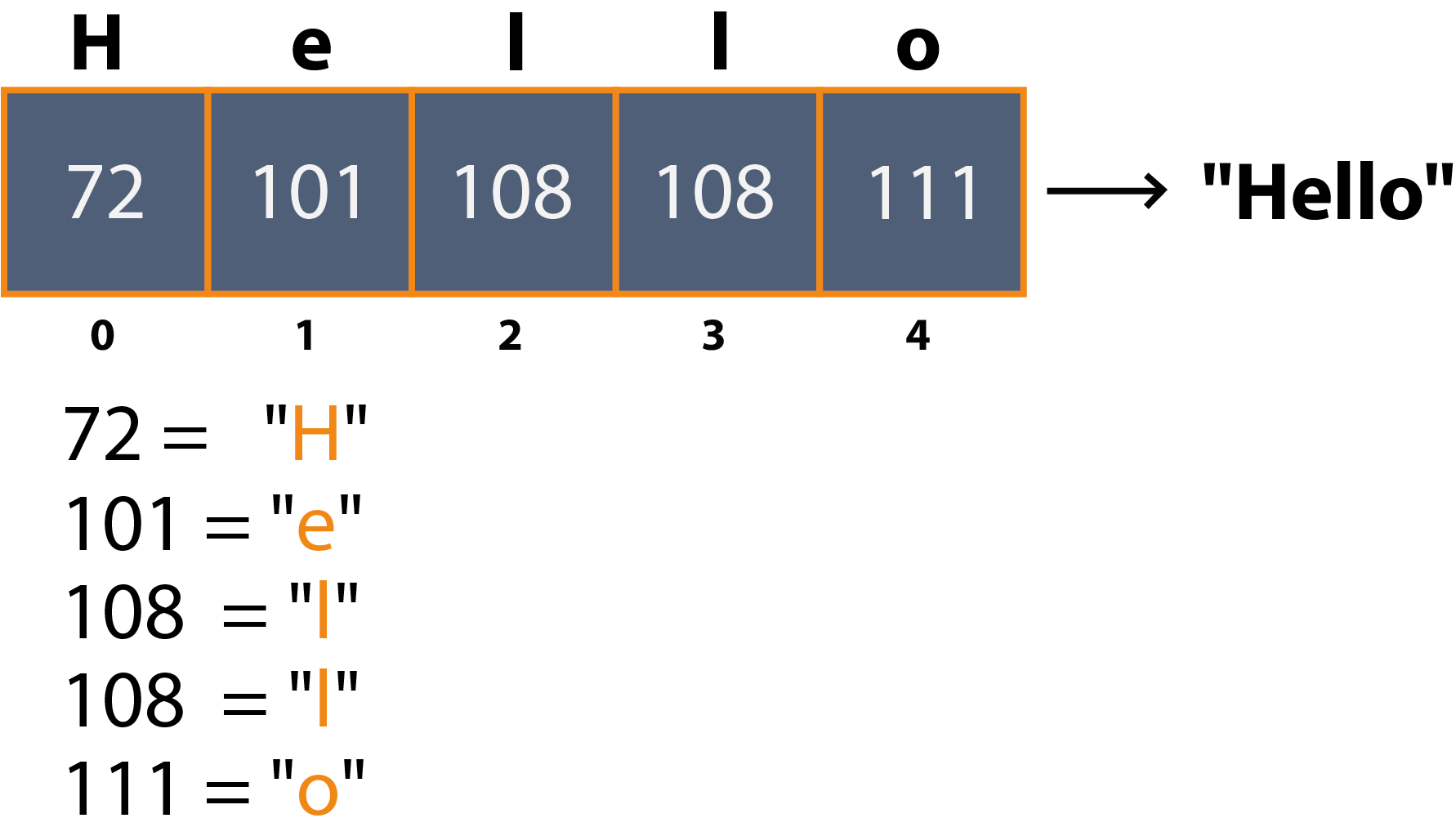Course Content

Java Extended

##Deeper into String# How String actually works?

You already know what a `String` is and how to work with it. This chapter will cover the theoretical aspects of what lies inside a `String`.

Let's explore the underlying representation of `String` values in Java. Initially, `String` was implemented as an array of `char` values, which was straightforward and intuitive. However, it later transitioned to an array of `byte` values. Let's understand the rationale behind this change.

A `char` variable in Java occupies 4 bytes of memory, while a `byte` variable only requires 1 byte. By utilizing `byte` values, we can significantly reduce the memory footprint, utilizing only one-fourth of the space.

But how does a `String` accommodate various characters, including non-numeric ones? The mechanism is akin to that of a `char` variable. We leverage the ASCII table, where numerical data is mapped to specific characters, enabling the representation of a wide range of characters in a `String`.

Let's take a look at an illustration representing the `String` value "Hello":The cells contain type `byte` elements, which we take from the ASCII table. We can even view in the code the exact `byte` array that is stored in the value of a `String` variable. This can be done using the `getBytes()` method.

Let's take a look at an example code:Main.javaWe can see that the `byte` values are identical to those shown in the diagram above. Additionally, if you're interested, you can refer to the ASCII table and compare the code of each element with its corresponding value.

We can manipulate this `byte[]` array that we obtain from a `String` object.Everything was clear?

Section 3. Chapter 1